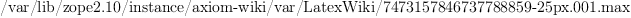login  home  contents  what's new  discussion  bug reports help  links  subscribe  changes  refresh  edit

# Edit detail for AboutMaxima revision 3 of 3

 1 2 3 Editor: Bill Page Time: 2009/01/07 09:34:11 GMT-8 Note: show version

added:
<pre>
\begin{maxima}
build_info()\$
\end{maxima}
</pre>



Maxima is a system for the manipulation of symbolic and numerical expressions, including differentiation, integration, Taylor series, Laplace transforms, ordinary differential equations, systems of linear equations, and vectors, matrices, and tensors. Maxima produces high precision results by using exact fractions and arbitrarily long floating point representations, and can plot functions and data in two and three dimensions.

Maxima is a descendant of Macsyma, the legendary computer algebra system developed in the late 1960s at the Massachusetts Institute of Technology. It is the only system based on that effort still publicly available and with an active user community, thanks to its open source nature. Macsyma was revolutionary in its day, and many later systems, such as Maple and Mathematica, were inspired by it.

For an introduction see Maxima Tutorial

RemoteWikiURL?: http://maxima.sourceforge.net

You can use Maxima on this website by writing:

  \begin{maxima}
maxima commands
\end{maxima}


and also via Sage, for example:

  \begin{sageblock}
fx=maxima('sin(x)')
fx.integrate('x')
\end{sageblock}



maxima(%i2) build_info()

(%o2)(1)



maxima(%i4) fx:sin(x)

maxima(%i5) integrate(fx,x)